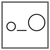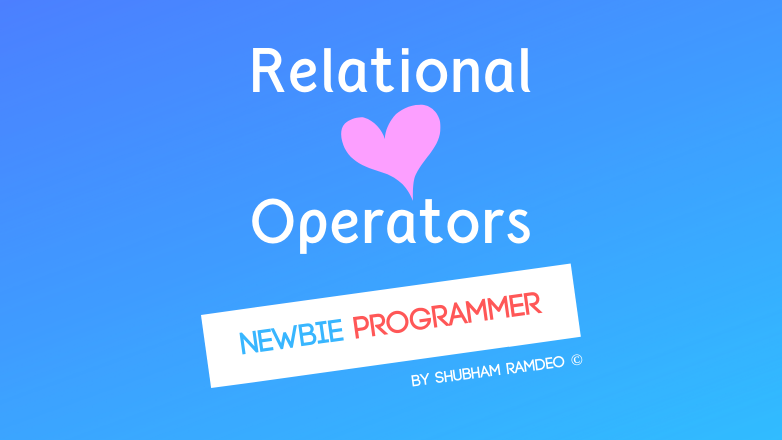Fuddlepixel coz tech is fun();

# Relational Operators

Let us discuss the relational operators through which we can perform relational comparison in the C programming language.

By Shubham Ramdeo
21 August 2015 · 5 mins read
# `c`  `newbie`How can a computer compares and measures ? It’s the Relational Operators !

Welcome back to the newbie programmer series. In the last post ( click here ), we have learn how to use the arithmetic operators. We have learn many tips and tricks to make our programming faster. In this part, we will learn about the relational operators. There are some you know, like < and > but this one will introduce more and will have more tricks and tips. So that we can make our program’s logic more effective. If you are new to this series, please go to the index ( click here ) and check out all the previous posts so that you can easily understand what is going down below.

The Relational operators defines the relations between two given data ( numbers ). The operations are if two numbers are equal, greater than, less than etc.

### The mathematics behind Relations

There is no middle. The simple formula a computer can understand is that If the relation is correct, i.e true, we get 1. The computer has its numerical conventions. So for it, 1 is true. So for example,

(5 > 2) means 1 for computer as its true. It’s not the value of 5 > 2, it’s just the Computer’s emotions I would say !!!

#### Need

We need relational operators so that the Computer can make decisions and so we can control the flow of the program.  Like as we did with the loops. If a computer cannot if a condition is true or false, then how will we be able to control the flow of the loops and yet we have discussed loops later we are gonna discuss the “ if then else” like statements and for controlling such things, relational operators are necessary.

### The Operators:

Remember how to use the operators. Its like :

left operand Operator right operand

And if the condition of the operand is satisfied, it will get a “true” or 1 actually. Instead of getting a result (answer) with we get using arithmetic operators like 2+5=7, here the result (answer) is either true or false.

Lets discuss all the relational operators one by one.

#### Less than “ < “

To compare if the left operand is less than the right operand.

( 5 < 8 ) is true

( 6 < 1 ) is not true

#### Greater than “ > “

To compare if the left operand is greater than the right operand.

( 2 > 1 ) is true

( 9 > 21 ) is not true

#### Less than or equal to “ <= “

To compare if the left operand is less than or equal to the right operand.

( 5 <= 8 ) is true

( 5 <= 5 ) is also true

( 5 <= 4 ) is not true

#### Greater than or equal to “ >= “

To compare if the left operand is greater than or equal to the right operand.

( 9 >= 1 ) is true

( 9 >= 9 ) is also true

( 9 >= 21 ) is not true

#### Equal to “ == “

To check if the left operand is numerically equal to the right operand.

( 6 == 6 ) is true

( 4 == 3 ) is not true

#### Difference between assignment ( = ) and equal to ( == )

The assignment operator is a single = sign. It assigns one value to a variable. Or value of a variable to another variable. But the equal to relational operators check if they are equal.

Suppose A = 5 and B = 10.

If I say A = B,  it means now A is 10

If I say A == B, it means “ NOT TRUE” as 5 is not equal to 10

So you got the difference !

#### Not equal to “ != “

To check if the left operand is numerically not equal to the right operand. Its simply reverse of “equal to” operator.

( 6 != 6 ) is NOT TRUE

( 6 != 3 ) is True

### Example:

These above were all the Relational Operators. Now we need to do an example to see all these practically. I have designed a great program that will help you check each of the above operators very easily.

``````#include <stdio.h>
main()
{
printf("Relation checker \n");

//change the value of variables
int A = 1;
int B = 2;
while ( A < B ) //change the operator
{
printf("It is correct ! \n");
return(0); //to exit
}
printf("Not correct \n");
return(0); //to exit
}
``````

How to use it ? Simple. Change the value of the variables as you want. Change the operator in the while loop to that one you want to check. If the relation between A and B which you have set is TRUE, the while loop will work and you will get printed “It is correct !” and then the program will exit. If the relation is NOT TRUE, the while loop will be skipped. You will get printed “Not Correct” and the program will exit.

That’s it for today. You can make your own programs and then send me. I will post your programs for you. Play more with the above operators. I will meet you in the next post with Logical Operators ! Stay Connected. Any doubts ? Meet me on twitter @ramdeoshubham

##### Update me weekly ✉

Subscribe below and we’ll send you a weekly email summary of all new Code tutorials. Never miss out on learning about the next big thing.

#### Shubham RamdeoShubham Ramdeo is the founder of Fuddlepixel. He is a geek, a writer, involved with many technical and humanity projects.

Meet me!

#### Recent#### Related

`c`

`newbie`

Top